# How to use the XLOOKUP function in ExcelWhen analyzing large amounts of data within a table or range in Excel, it is a common practice to track certain values and find the corresponding results. Microsoft’s XLOOKUP function in excel is one of the many functions that you can use for a range of search types of your data.

XLOOKUP is the function you need when want to search for values by row. It allows you to select your desired range of data across multiple columns in order to find the corresponding value or values in the same row. The XLOOKUP function can also provide the closest approximate match if it fails to find the specific value you are looking for. This makes it the most powerful function of the excel functions available, due to its versatility in searches and approximation abilities.

Let’s take a look at what makes up the XLOOKUP function and how you can use this function in Excel.

## Syntax

The following are the two versions of XLOOKUP you can use to search for data.

=XLOOKUP(lookup_value, lookup_array, return_array)

• lookup_value is the value to search for
• lookup_array is the range of data to search from
• return_array is the array of data to return, in other words, the data you’re looking for

To include approximate matches if your exact value isn’t found, use the following formula instead.

=XLOOKUP(lookup_value, lookup_array, return_array, [if_not_found], [match_mode], [search_mode])

• [if_not_found] is the determined value if an exact match isn’t found. For example, setting this to ‘0’ means that if the formula can’t find a match, it will display a ‘0’ instead.
• [match_mode] indicates how accurate this match is set to be.
0 = if no exact match, no alternative
-1 = if no exact match, next smallest item
1 = if no exact match, next largest item
2 = wildcard match
• [search_mode] specifies the type of search to use.
1 = search starting from the first item in your table
-1 = reverse search starting from the last item in your table
2 = binary search of the lookup_array that is in ascending order. (this must be correct in order to function properly)
-2 = binary search of the lookup_array that is in descending order. (this must be correct in order to function properly)

### How to use the XLOOKUP function in Excel

Now let’s put the above into practice. To demonstrate how the XLOOKUP function in Excel works, we will use the case study of identifying the sales and number of employees of specific retail departments.

First, let’s start with identifying this month’s sales of various departments using our XLOOKUP function.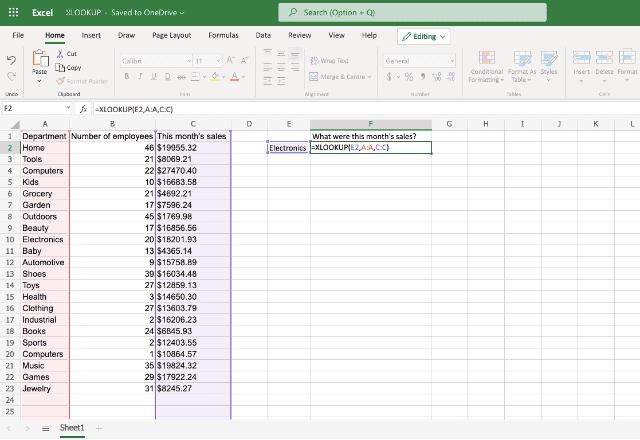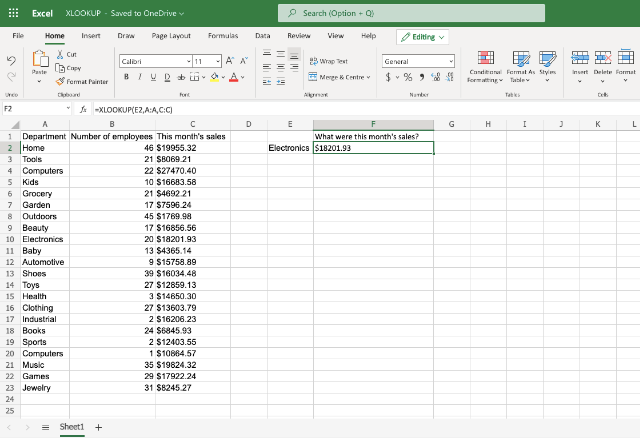In order to find this month’s sales in the Electronics department, I have carried out the following steps:

1. Dedicate a cell to where I want my information to be located (including the name of the department next to this).
2. Start creating the function with =XLOOKUP (and selecting the ‘Electronics’ cell (E2) I created. This is the lookup_value I want to find within the table of data.
3. After highlighting the lookup_value, add a comma. Then select the A column where my department data is located – this is the lookup_array in which the ‘Electronics’ department will be searched for.
4. Add another comma after this lookup_array. Then highlight the column where the value I’m looking for is located (column C). Finish the XLOOKUP function by adding a final bracket ‘ ) ‘.
5. The finished formula looks like this =XLOOKUP(E2,A:A,C:C). Press ‘enter’ on your keyboard for the formula to find your value.

As you can see, following these steps, the XLOOKUP formula has correctly provided this month’s sales for the electronics department.

In order to find another department’s sales data, we can simply replace the text in cell E2 to another department name such as ‘Books’. The excel will automatically update with the new sales data.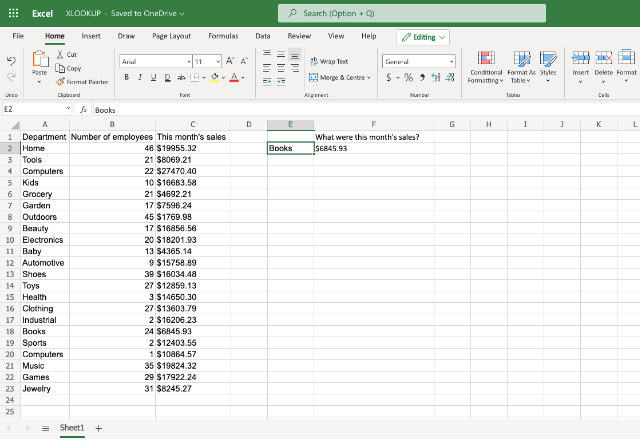We can also return multiple items of data by changing the lookup_array to cover more than one column.

For example, if I wanted to find both the number of employees and this month’s sales for the Grocery department, the XLOOKUP formula would look like this =XLOOKUP(E2,A:A,B:C).

After pressing ‘enter’, both values are correctly displayed.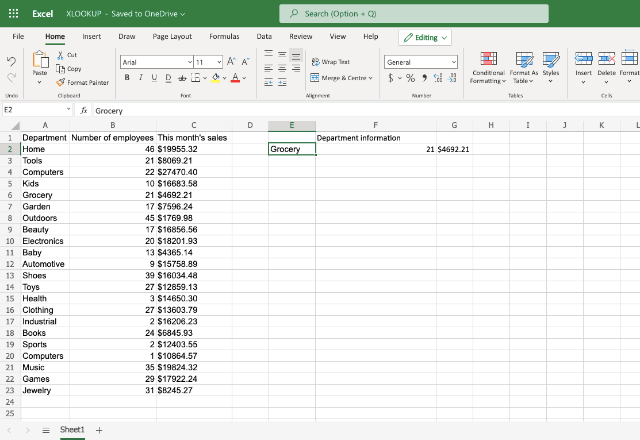The ability to search for values in two different columns is something that is completely unique to the XLOOKUP formula. This is why XLOOKUP is a great option for those wanting to find various values in a big data sheet with ease – it can only be done using the XLOOKUP formula.

Finally, let’s look for values using the optional XLOOKUP function to find an approximate match. For this case study, let’s use another table of data and try to find the maximum number of employees needed to make a set amount of money.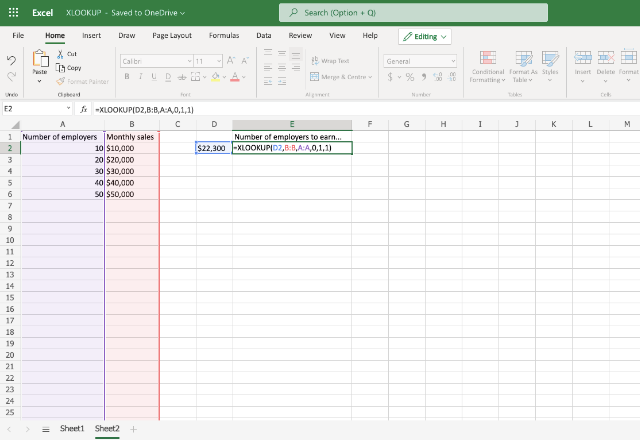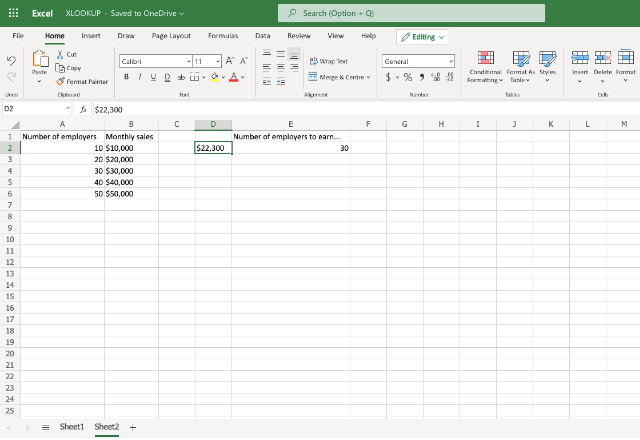Here are the steps following to use the XLOOKUP formula to show the maximum number of employees needed to make \$22,300.

1. First, we start with the required function we have talked about in the other examples =XLOOKUP(D2,B:B,A:A.
2. Following this, add another comma, and set your [if_not_value] – here I have established it as ‘0’.
3. Then, choose the accuracy of the match. As we are looking for the maximum number of employees, I have set this to ‘1’. This should provide us with the next largest match.
4. Add another comma. Now, choose the search type – we can use the normal search type here, so add ‘1’. End your XLOOKUP formula with a final bracket – ‘)’.
5. Your final XLOOKUP formula should look like this =XLOOKUP(D2,B:B,A:A,0,1,1).

As you can see, the XLOOKUP formula has provided us with the correct value for the maximum number of employees we’d need to make \$22,300.

### XLOOKUP in Excel

And there you have it! The XLOOKUP function is an extremely powerful formula that can help you carry out a variety of data searches with intuitive matching abilities.

To find out more about other LOOKUP functions within excel, check out our articles below.